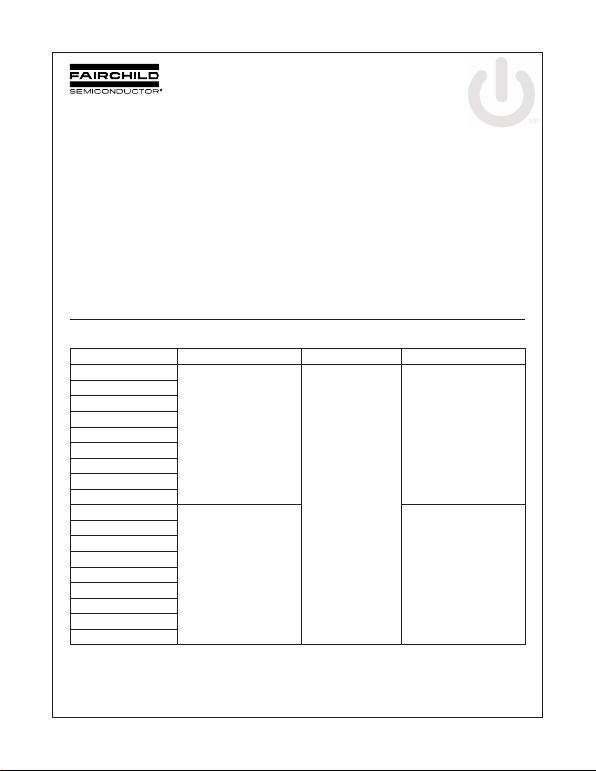1
www.fairchildsemi.com
LM78XX/LM78XXA Rev. 1.0.1
LM78XX/LM78XXA 3-Terminal 1A Positive Voltage Regulator
May 2006
LM78XX/LM78XXA
3-Terminal 1A Positive Voltage Regulator
Features
Output Current up to 1A
Output Voltages of 5, 6, 8, 9, 10, 12, 15, 18, 24
Short Circuit Protection
Output Transistor Safe Operating Area Protection
General Description
The LM78XX series of three terminal positive regulators
are available in the TO-220 package and with several
ﬁxed output voltages, making them useful in a wide
range of applications. Each type employs internal current
limiting, thermal shut down and safe operating area pro-
tection, making it essentially indestructible. If adequate
heat sinking is provided, they can deliver over 1A output
current. Although designed primarily as ﬁxed voltage
regulators, these devices can be used with external com-
ponents to obtain adjustable voltages and currents.
Ordering Information
Product Number Output Voltage Tolerance Package Operating Temperature
LM7805CT
±
4% TO-220 -40°C to +125°C
LM7806CT
LM7808CT
LM7809CT
LM7810CT
LM7812CT
LM7815CT
LM7818CT
LM7824CT
LM7805ACT
±
2% 0°C to +125°C
LM7806ACT
LM7808ACT
LM7809ACT
LM7810ACT
LM7812ACT
LM7815ACT
LM7818ACT
LM7824ACT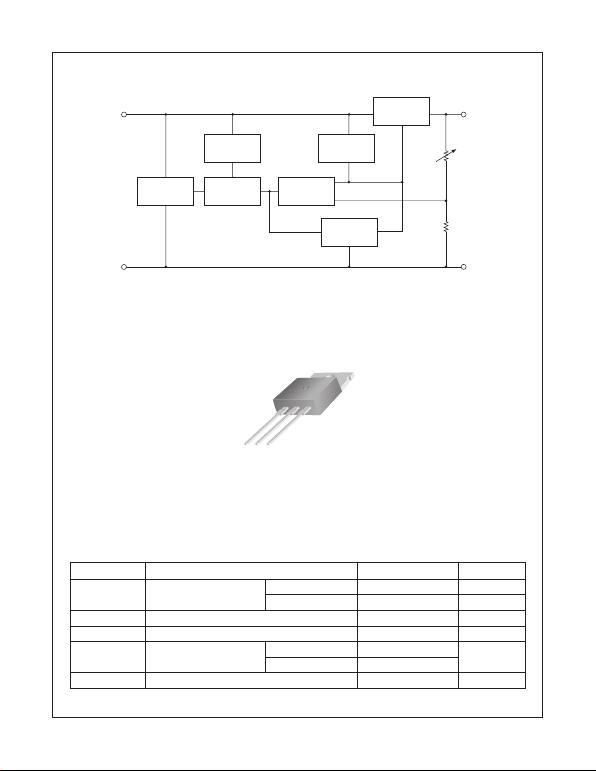2
www.fairchildsemi.com
LM78XX/LM78XXA Rev. 1.0.1
LM78XX/LM78XXA 3-Terminal 1A Positive Voltage Regulator
Block Diagram
Figure 1.
Pin Assignment
Figure 2.
Absolute Maximum Ratings
Absolute maximum ratings are those values beyond which damage to the device may occur. The datasheet
speciﬁcations should be met, without exception, to ensure that the system design is reliable over its power supply,
temperature, and output/input loading variables. Fairchild does not recommend operation outside datasheet
speciﬁcations.
Symbol Parameter Value Unit
V
I
Input Voltage V
O
= 5V to 18V 35 V
V
O
= 24V 40 V
R
θ
JC
Thermal Resistance Junction-Cases (TO-220) 5 °C/W
R
θ
JA
Thermal Resistance Junction-Air (TO-220) 65 °C/W
T
OPR
Operating Temperature
Range
LM78xx -40 to +125 °C
LM78xxA 0 to +125
T
STG
Storage Temperature Range -65 to +150 °C
Starting
Circuit
Input
1
Reference
Voltage
Current
Generator
SOA
Protection
Thermal
Protection
Series Pass
Element
Error
Amplifier
Output
3
GND
2
1
1. Input
2. GND
3. Output
GND
TO-220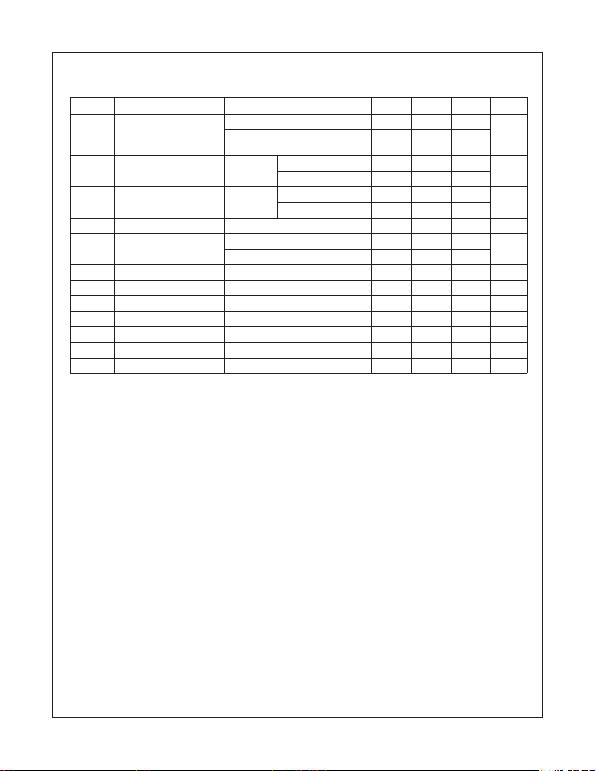3
www.fairchildsemi.com
LM78XX/LM78XXA Rev. 1.0.1
LM78XX/LM78XXA 3-Terminal 1A Positive Voltage Regulator
Electrical Characteristics (LM7805)
Refer to the test circuits. -40°C
<
T
J
<
125°C, I
O
= 500mA, V
I
= 10V, C
I
= 0.1
µ
F, unless otherwise speciﬁed.
Notes:
1. Load and line regulation are speciﬁed at constant junction temperature. Changes in V
O
due to heating effects must
be taken into account separately. Pulse testing with low duty is used.
2. These parameters, although guaranteed, are not 100% tested in production.
Symbol Parameter Conditions Min. Typ. Max. Unit
V
O
Output Voltage T
J
= +25°C 4.8 5.0 5.2 V
5mA
I
O
1A, P
O
15W,
V
I
= 7V to 20V
4.75 5.0 5.25
Regline Line Regulation
(1)
T
J
= +25°C V
O
= 7V to 25V 4.0 100 mV
V
I
= 8V to 12V 1.6 50.0
(1)
T
J
= +25°C I
O
= 5mA to 1.5A 9.0 100 mV
I
O
= 250mA to 750mA 4.0 50.0
I
Q
Quiescent Current T
J
= +25°C 5.0 8.0 mA
I
Q
Quiescent Current Change I
O
= 5mA to 1A 0.03 0.5 mA
V
I
= 7V to 25V 0.3 1.3
V
O
/
TOutput Voltage Drift
(2)
I
O
= 5mA -0.8 mV/°C
V
N
Output Noise Voltage f = 10Hz to 100kHz, T
A
= +25°C 42.0
µ
V/V
O
RR Ripple Rejection
(2)
f = 120Hz, V
O
= 8V to 18V 62.0 73.0 dB
V
DROP
Dropout Voltage I
O
= 1A, T
J
= +25°C 2.0 V
r
O
Output Resistance
(2)
f = 1kHz 15.0 m
I
SC
Short Circuit Current V
I
= 35V, T
A
= +25°C 230 mA
I
PK
Peak Current
(2)
T
J
= +25°C 2.2 A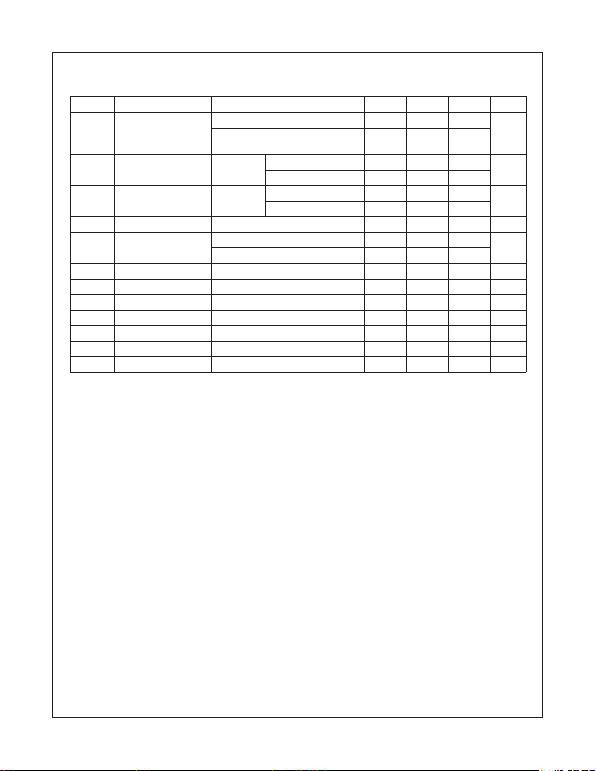4
www.fairchildsemi.com
LM78XX/LM78XXA Rev. 1.0.1
LM78XX/LM78XXA 3-Terminal 1A Positive Voltage Regulator
Electrical Characteristics (LM7806)
(Continued)
Refer to the test circuits. -40°C
<
T
J
<
125°C, I
O
= 500mA, V
I
= 11V, C
I
= 0.33
µ
F, C
O
= 0.1
µ
F, unless otherwise speciﬁed.
Notes:
3. Load and line regulation are speciﬁed at constant junction temperature. Changes in V
O
due to heating effects must
be taken into account separately. Pulse testing with low duty is used.
4. These parameters, although guaranteed, are not 100% tested in production.
Symbol Parameter Conditions Min Typ. Max. Unit
V
O
Output Voltage T
J
= +25°C 5.75 6.0 6.25 V
5mA
I
O
1A, P
O
15W,
V
I
= 8.0V to 21V
5.7 6.0 6.3
Regline Line Regulation
(3)
T
J
= +25°C V
I
= 8V to 25V 5.0 120 mV
V
I
= 9V to 13V 1.5 60.0
(3)
T
J
= +25°C I
O
= 5mA to 1.5A 9.0 120 mV
I
O
= 250mA to 750mA 3.0 60.0
I
Q
Quiescent Current T
J
= +25°C 5.0 8.0 mA
I
Q
Quiescent Current
Change
I
O
= 5mA to 1A 0.5 mA
V
I
= 8V to 25V 1.3
V
O
/
TOutput Voltage Drift
(4)
I
O
= 5mA -0.8 mV/°C
V
N
Output Noise Voltage f = 10Hz to 100kHz, T
A
= +25°C 45.0
µ
V/V
O
RR Ripple Rejection
(4)
f = 120Hz, V
O
= 8V to 18V 62.0 73.0 dB
VDROP Dropout Voltage IO = 1A, TJ = +25°C 2.0 V
rOOutput Resistance(4) f = 1kHz 19.0 m
ISC Short Circuit Current VI = 35V, TA = +25°C 250 mA
IPK Peak Current(4) TJ = +25°C 2.2 A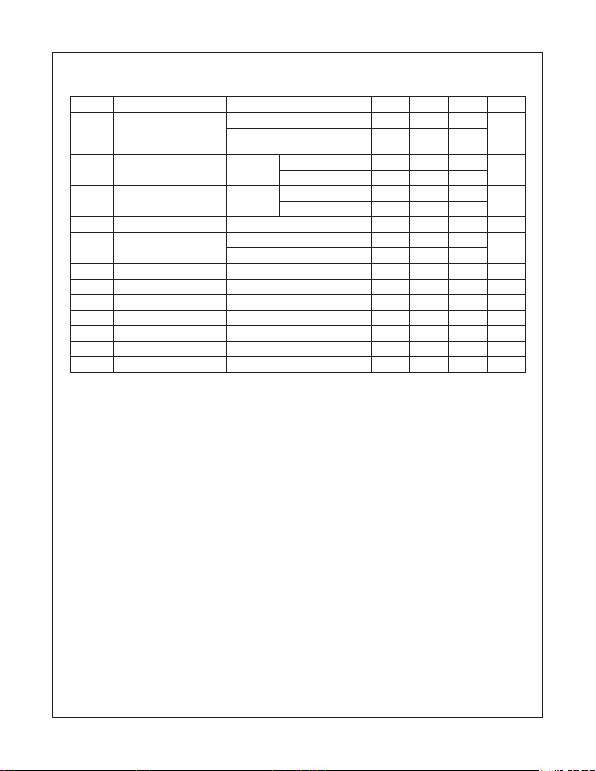5www.fairchildsemi.com
LM78XX/LM78XXA Rev. 1.0.1
LM78XX/LM78XXA 3-Terminal 1A Positive Voltage Regulator
Electrical Characteristics (LM7808) (Continued)
Refer to the test circuits. -40°C < TJ < 125°C, IO = 500mA, VI = 14V, CI = 0.33µF, C O = 0.1µF, unless otherwise speciﬁed.
Notes:
5. Load and line regulation are speciﬁed at constant junction temperature. Changes in VO due to heating effects must
be taken into account separately. Pulse testing with low duty is used.
6. These parameters, although guaranteed, are not 100% tested in production.
Symbol Parameter Conditions Min. Typ. Max. Unit
VOOutput Voltage TJ = +25°C 7.7 8.0 8.3 V
5mA IO 1A, PO 15W,
VI = 10.5V to 23V
7.6 8.0 8.4
Regline Line Regulation(5) TJ = +25°C VI = 10.5V to 25V 5.0 160 mV
VI = 11.5V to 17V 2.0 80.0
Regload Load Regulation(5) TJ = +25°C IO = 5mA to 1.5A 10.0 160 mV
IO = 250mA to 750mA 5.0 80.0
IQQuiescent Current TJ = +25°C 5.0 8.0 mA
IQQuiescent Current Change IO = 5mA to 1A 0.05 0.5 mA
VI = 10.5V to 25V 0.5 1.0
VO/TOutput Voltage Drift(6) IO = 5mA -0.8 mV/°C
VNOutput Noise Voltage f = 10Hz to 100kHz, T
A = +25°C 52.0 µV/VO
RR Ripple Rejection(6) f = 120Hz, VO = 11.5V to 21.5V 56.0 73.0 dB
VDROP Dropout Voltage IO = 1A, TJ = +25°C 2.0 V
rOOutput Resistance(6) f = 1kHz 17.0 m
ISC Short Circuit Current VI = 35V, TA = +25°C 230 mA
IPK Peak Current(6) TJ = +25°C 2.2 A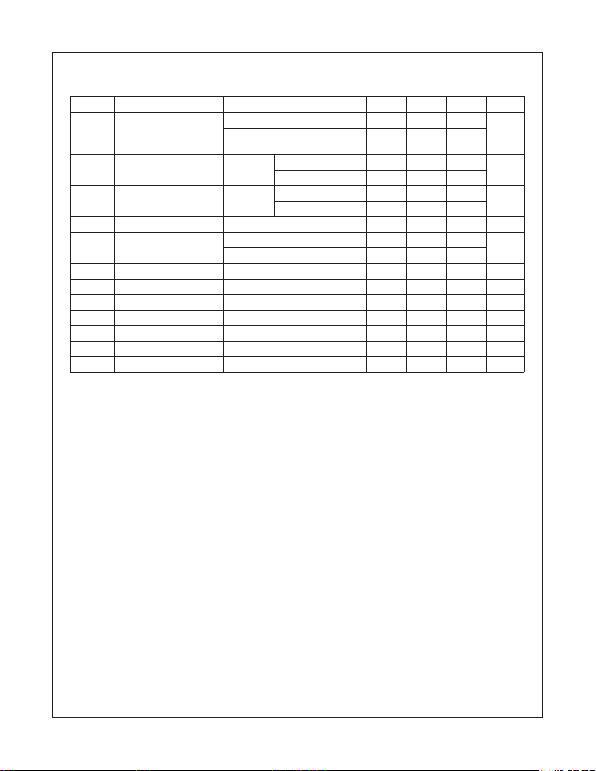6www.fairchildsemi.com
LM78XX/LM78XXA Rev. 1.0.1
LM78XX/LM78XXA 3-Terminal 1A Positive Voltage Regulator
Electrical Characteristics (LM7809) (Continued)
Refer to the test circuits. -40°C < TJ < 125°C, IO = 500mA, VI = 15V, CI = 0.33µF, C O = 0.1µF, unless otherwise speciﬁed.
Notes:
7. Load and line regulation are speciﬁed at constant junction temperature. Changes in VO due to heating effects must
be taken into account separately. Pulse testing with low duty is used.
8. These parameters, although guaranteed, are not 100% tested in production.
Symbol Parameter Conditions Min. Typ. Max. Unit
VOOutput Voltage TJ = +25°C 8.65 9.0 9.35 V
5mA IO 1A, PO 15W,
VI = 11.5V to 24V
8.6 9.0 9.4
Regline Line Regulation(7) TJ = +25°C VI = 11.5V to 25V 6.0 180 mV
VI = 12V to 17V 2.0 90.0
J = +25°C IO = 5mA to 1.5A 12.0 180 mV
IO = 250mA to 750mA 4.0 90.0
IQQuiescent Current TJ = +25°C 5.0 8.0 mA
IQQuiescent Current Change IO = 5mA to 1A 0.5 mA
VI = 11.5V to 26V 1.3
VO/TOutput Voltage Drift(8) IO = 5mA -1.0 mV/°C
VNOutput Noise Voltage f = 10Hz to 100kHz, T
A = +25°C 58.0 µV/VO
RR Ripple Rejection(8) f = 120Hz, VO = 13V to 23V 56.0 71.0 dB
VDROP Dropout Voltage IO = 1A, TJ = +25°C 2.0 V
rOOutput Resistance(8) f = 1kHz 17.0 m
ISC Short Circuit Current VI = 35V, TA = +25°C 250 mA
IPK Peak Current(8) TJ = +25°C 2.2 A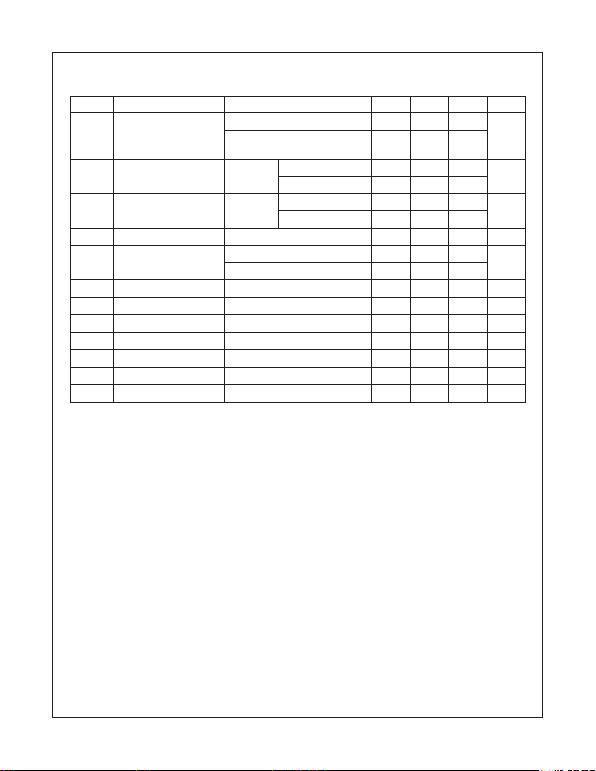7www.fairchildsemi.com
LM78XX/LM78XXA Rev. 1.0.1
LM78XX/LM78XXA 3-Terminal 1A Positive Voltage Regulator
Electrical Characteristics (LM7810) (Continued)
Refer to the test circuits. -40°C < TJ < 125°C, IO = 500mA, VI = 16V, CI = 0.33µF, C O = 0.1µF, unless otherwise speciﬁed.
Notes:
9. Load and line regulation are speciﬁed at constant junction temperature. Changes in VO due to heating effects must
be taken into account separately. Pulse testing with low duty is used.
10. These parameters, although guaranteed, are not 100% tested in production.
Symbol Parameter Conditions Min. Typ. Max. Unit
VOOutput Voltage TJ = +25°C 9.6 10.0 10.4 V
5mA IO 1A, PO 15W,
VI = 12.5V to 25V
9.5 10.0 10.5
Regline Line Regulation(9) TJ = +25°C VI = 12.5V to 25V 10.0 200 mV
VI = 13V to 25V 3.0 100
Regload Load Regulation(9) TJ = +25°C IO = 5mA to 1.5A 12.0 200 mV
IO = 250mA to 750mA 4.0 400
IQQuiescent Current TJ = +25°C 5.1 8.0 mA
IQQuiescent Current Change IO = 5mA to 1A 0.5 mA
VI = 12.5V to 29V 1.0
VO/TOutput Voltage Drift(10) IO = 5mA -1.0 mV/°C
VNOutput Noise Voltage f = 10Hz to 100kHz, T
A = +25°C 58.0 µV/VO
RR Ripple Rejection(10) f = 120Hz, VO = 13V to 23V 56.0 71.0 dB
VDROP Dropout Voltage IO = 1A, TJ = +25°C 2.0 V
rOOutput Resistance(10) f = 1kHz 17.0 m
ISC Short Circuit Current VI = 35V, TA = +25°C 250 mA
IPK Peak Current(10) TJ = +25°C 2.2 A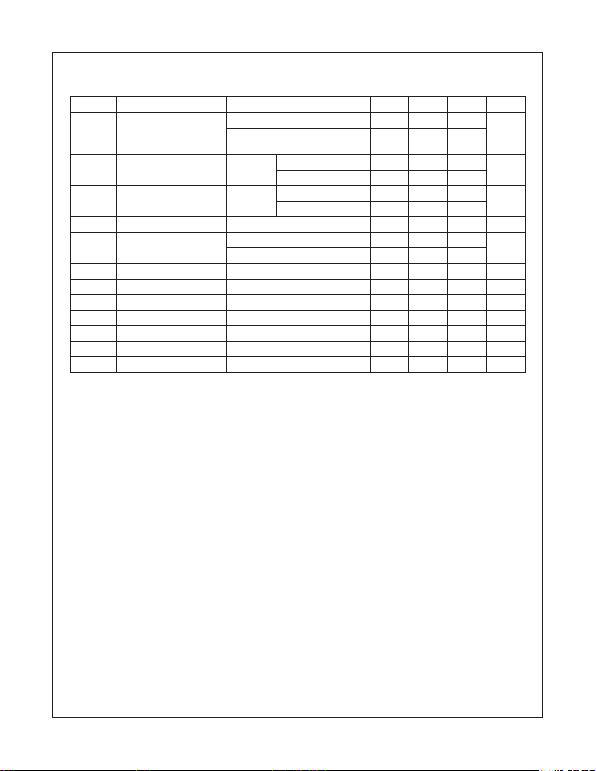8www.fairchildsemi.com
LM78XX/LM78XXA Rev. 1.0.1
LM78XX/LM78XXA 3-Terminal 1A Positive Voltage Regulator
Electrical Characteristics (LM7812) (Continued)
Refer to the test circuits. -40°C < TJ < 125°C, IO = 500mA, VI = 19V, CI = 0.33µF, C O = 0.1µF, unless otherwise speciﬁed.
Notes:
11. Load and line regulation are speciﬁed at constant junction temperature. Changes in VO due to heating effects must
be taken into account separately. Pulse testing with low duty is used.
12. These parameters, although guaranteed, are not 100% tested in production.
Symbol Parameter Conditions Min. Typ. Max. Unit
VOOutput Voltage TJ = +25°C 11.5 12.0 12.5 V
5mA IO 1A, PO 15W,
VI = 14.5V to 27V
11.4 12.0 12.6
Regline Line Regulation(11) TJ = +25°C VI = 14.5V to 30V 10.0 240 mV
VI = 16V to 22V 3.0 120
Regload Load Regulation(11) TJ = +25°C IO = 5mA to 1.5A 11.0 240 mV
IO = 250mA to 750mA 5.0 120
IQQuiescent Current TJ = +25°C 5.1 8.0 mA
IQQuiescent Current Change IO = 5mA to 1A 0.1 0.5 mA
VI = 14.5V to 30V 0.5 1.0
VO/TOutput Voltage Drift(12) IO = 5mA -1.0 mV/°C
VNOutput Noise Voltage f = 10Hz to 100kHz, T
A = +25°C 76.0 µV/VO
RR Ripple Rejection(12) f = 120Hz, VI = 15V to 25V 55.0 71.0 dB
VDROP Dropout Voltage IO = 1A, TJ = +25°C 2.0 V
rOOutput Resistance(12) f = 1kHz 18.0 m
ISC Short Circuit Current VI = 35V, TA = +25°C 230 mA
IPK Peak Current(12) TJ = +25°C 2.2 A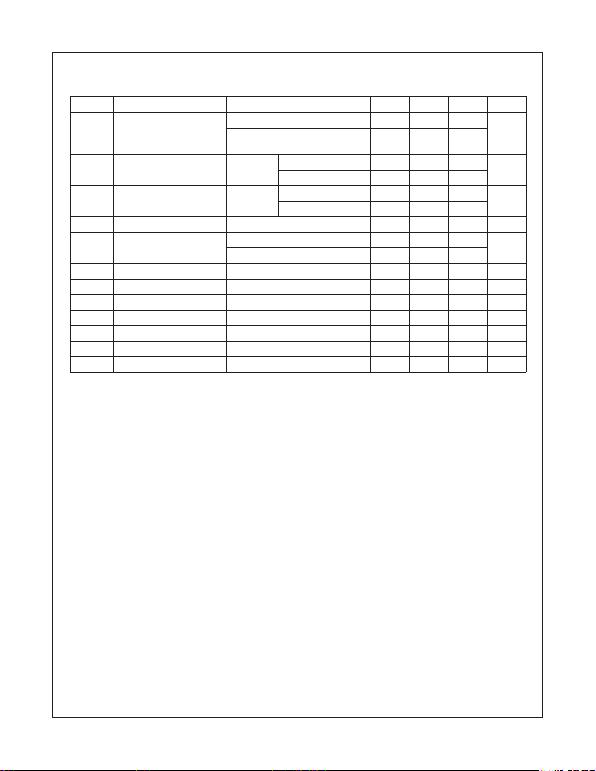9www.fairchildsemi.com
LM78XX/LM78XXA Rev. 1.0.1
LM78XX/LM78XXA 3-Terminal 1A Positive Voltage Regulator
Electrical Characteristics (LM7815) (Continued)
Refer to the test circuits. -40°C < TJ < 125°C, IO = 500mA, VI = 23V, CI = 0.33µF, C O = 0.1µF, unless otherwise speciﬁed.
Notes:
13. Load and line regulation are speciﬁed at constant junction temperature. Changes in VO due to heating effects must
be taken into account separately. Pulse testing with low duty is used.
14. These parameters, although guaranteed, are not 100% tested in production.
Symbol Parameter Conditions Min. Typ. Max. Unit
VOOutput Voltage TJ = +25°C 14.4 15.0 15.6 V
5mA IO 1A, PO 15W,
VI = 17.5V to 30V
14.25 15.0 15.75
Regline Line Regulation(13) TJ = +25°C VI = 17.5V to 30V 11.0 300 mV
VI = 20V to 26V 3.0 150
Regload Load Regulation(13) TJ = +25°C IO = 5mA to 1.5A 12.0 300 mV
IO = 250mA to 750mA 4.0 150
IQQuiescent Current TJ = +25°C 5.2 8.0 mA
IQQuiescent Current Change IO = 5mA to 1A 0.5 mA
VI = 17.5V to 30V 1.0
VO/TOutput Voltage Drift(14) IO = 5mA -1.0 mV/°C
VNOutput Noise Voltage f = 10Hz to 100kHz, T
A = +25°C 90.0 µV/VO
RR Ripple Rejection(14) f = 120Hz, VI = 18.5V to 28.5V 54.0 70.0 dB
VDROP Dropout Voltage IO = 1A, TJ = +25°C 2.0 V
rOOutput Resistance(14) f = 1kHz 19.0 m
ISC Short Circuit Current VI = 35V, TA = +25°C 250 mA
IPK Peak Current(14) TJ = +25°C 2.2 A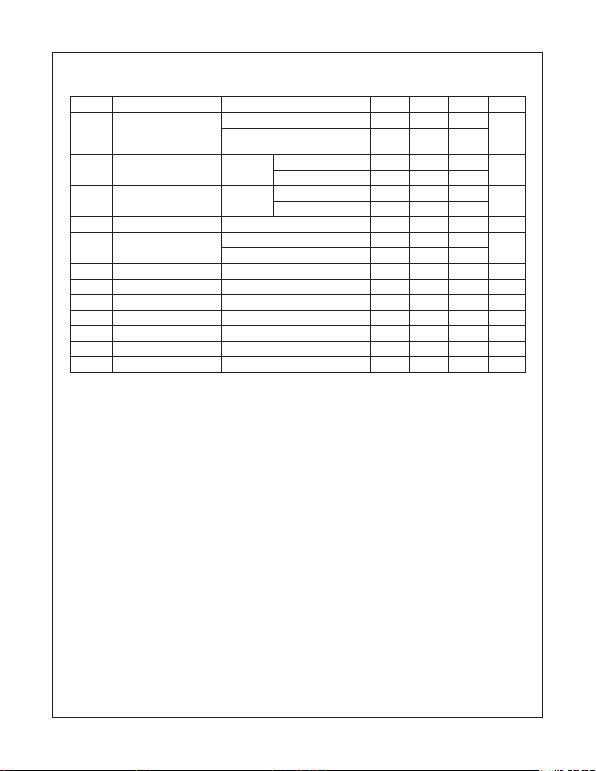10 www.fairchildsemi.com
LM78XX/LM78XXA Rev. 1.0.1
LM78XX/LM78XXA 3-Terminal 1A Positive Voltage Regulator
Electrical Characteristics (LM7818) (Continued)
Refer to the test circuits. -40°C < TJ < 125°C, IO = 500mA, VI = 27V, CI = 0.33µF, C O = 0.1µF, unless otherwise speciﬁed.
Notes:
15. Load and line regulation are speciﬁed at constant junction temperature. Changes in VO due to heating effects must
be taken into account separately. Pulse testing with low duty is used.
16. These parameters, although guaranteed, are not 100% tested in production.
Symbol Parameter Conditions Min. Typ. Max. Unit
VOOutput Voltage TJ = +25°C 17.3 18.0 18.7 V
5mA IO 1A, PO 15W,
VI = 21V to 33V
17.1 18.0 18.9
Regline Line Regulation(15) TJ = +25°C VI = 21V to 33V 15.0 360 mV
VI = 24V to 30V 5.0 180
Regload Load Regulation(15) TJ = +25°C IO = 5mA to 1.5A 15.0 360 mV
IO = 250mA to 750mA 5.0 180
IQQuiescent Current TJ = +25°C 5.2 8.0 mA
IQQuiescent Current Change IO = 5mA to 1A 0.5 mA
VI = 21V to 33V 1.0
VO/TOutput Voltage Drift(16) IO = 5mA -1.0 mV/°C
VNOutput Noise Voltage f = 10Hz to 100kHz, T
A = +25°C 110 µV/VO
RR Ripple Rejection(16) f = 120Hz, VI = 22V to 32V 53.0 69.0 dB
VDROP Dropout Voltage IO = 1A, TJ = +25°C 2.0 V
rOOutput Resistance(16) f = 1kHz 22.0 m
ISC Short Circuit Current VI = 35V, TA = +25°C 250 mA
IPK Peak Current(16) TJ = +25°C 2.2 A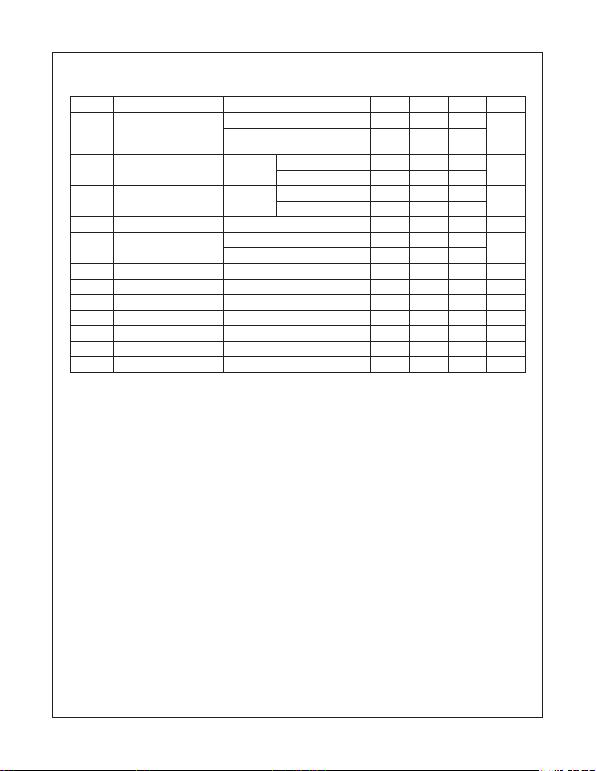11 www.fairchildsemi.com
LM78XX/LM78XXA Rev. 1.0.1
LM78XX/LM78XXA 3-Terminal 1A Positive Voltage Regulator
Electrical Characteristics (LM7824) (Continued)
Refer to the test circuits. -40°C < TJ < 125°C, IO = 500mA, VI = 33V, CI = 0.33µF, C O = 0.1µF, unless otherwise speciﬁed.
Notes:
17. Load and line regulation are speciﬁed at constant junction temperature. Changes in VO due to heating effects must
be taken into account separately. Pulse testing with low duty is used.
18. These parameters, although guaranteed, are not 100% tested in production.
Symbol Parameter Conditions Min. Typ. Max. Unit
VOOutput Voltage TJ = +25°C 23.0 24.0 25.0 V
5mA IO 1A, PO 15W,
VI = 27V to 38V
22.8 24.0 25.25
Regline Line Regulation(17) TJ = +25°C VI = 27V to 38V 17.0 480 mV
VI = 30V to 36V 6.0 240
Regload Load Regulation(17) TJ = +25°C IO = 5mA to 1.5A 15.0 480 mV
IO = 250mA to 750mA 5.0 240
IQQuiescent Current TJ = +25°C 5.2 8.0 mA
IQQuiescent Current Change IO = 5mA to 1A 0.1 0.5 mA
VI = 27V to 38V 0.5 1.0
VO/TOutput Voltage Drift(18) IO = 5mA -1.5 mV/°C
VNOutput Noise Voltage f = 10Hz to 100kHz, T
A = +25°C 60.0 µV/VO
RR Ripple Rejection(18) f = 120Hz, VI = 28V to 38V 50.0 67.0 dB
VDROP Dropout Voltage IO = 1A, TJ = +25°C 2.0 V
rO Output Resistance(18) f = 1kHz 28.0 m
ISC Short Circuit Current VI = 35V, TA = +25°C 230 mA
IPK Peak Current(18) TJ = +25°C 2.2 A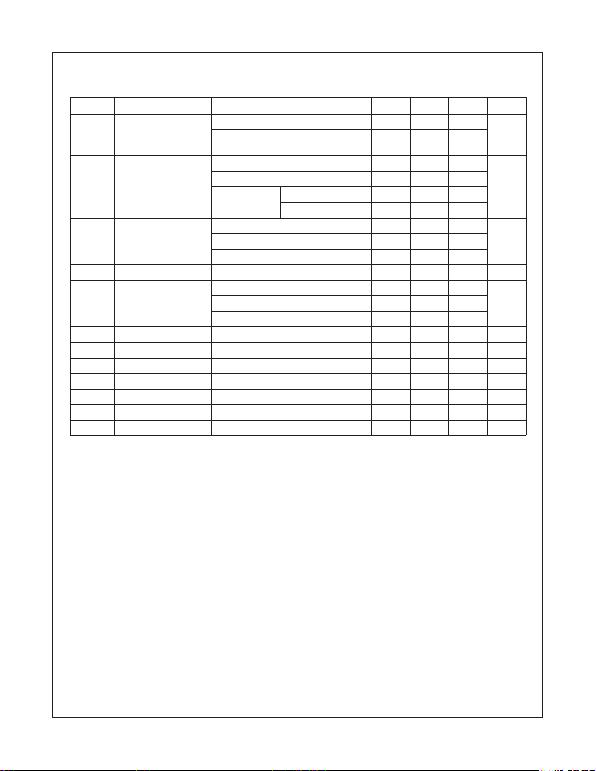12 www.fairchildsemi.com
LM78XX/LM78XXA Rev. 1.0.1
LM78XX/LM78XXA 3-Terminal 1A Positive Voltage Regulator
Electrical Characteristics (LM7805A) (Continued)
Refer to the test circuits. 0°C < TJ < 125°C, IO = 1A, VI = 10V, CI = 0.33µF, C O = 0.1µF, unless otherwise speciﬁed.
Notes:
19. Load and line regulation are speciﬁed at constant junction temperature. Changes in VO due to heating effects must
be taken into account separately. Pulse testing with low duty is used.
20. These parameters, although guaranteed, are not 100% tested in production.
Symbol Parameter Conditions Min. Typ. Max. Unit
VOOutput Voltage TJ = +25°C 4.9 5.0 5.1 V
IO = 5mA to 1A, PO 15W,
VI = 7.5V to 20V
4.8 5.0 5.2
Regline Line Regulation(19) VI = 7.5V to 25V, IO = 500mA 5.0 50.0 mV
VI = 8V to 12V 3.0 50.0
TJ = +25°C VI = 7.3V to 20V 5.0 50.0
VI = 8V to 12V 1.5 25.0
Regload Load Regulation(19) TJ = +25°C, IO = 5mA to 1.5A 9.0 100 mV
IO = 5mA to 1A 9.0 100
IO = 250mA to 750mA 4.0 50.0
IQQuiescent Current TJ = +25°C 5.0 6.0 mA
IQQuiescent Current
Change
IO = 5mA to 1A 0.5 mA
VI = 8V to 25V, IO = 500mA 0.8
VI = 7.5V to 20V, TJ = +25°C 0.8
VO/TOutput Voltage Drift(20) IO = 5mA -0.8 mV/°C
VNOutput Noise Voltage f = 10Hz to 100kHz, T
A = +25°C 10.0 µV/VO
RR Ripple Rejection(20) f = 120Hz, IO = 500mA, VI = 8V to 18V 68.0 dB
VDROP Dropout Voltage IO = 1A, TJ = +25°C 2.0 V
rOOutput Resistance(20) f = 1kHz 17.0 m
ISC Short Circuit Current VI = 35V, TA = +25°C 250 mA
IPK Peak Current(20) TJ = +25°C 2.2 A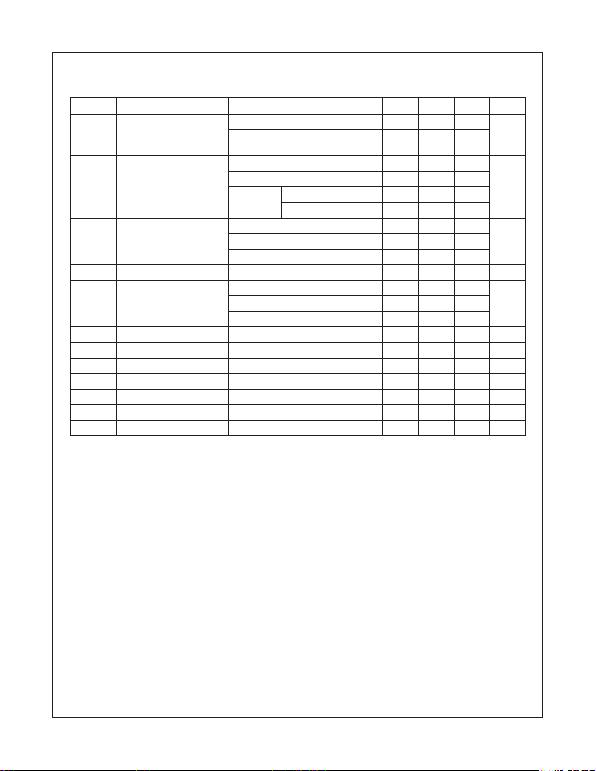13 www.fairchildsemi.com
LM78XX/LM78XXA Rev. 1.0.1
LM78XX/LM78XXA 3-Terminal 1A Positive Voltage Regulator
Electrical Characteristics (LM7806A) (Continued)
Refer to the test circuits. 0°C < TJ < 125°C, IO = 1A, VI = 11V, CI = 0.33µF, C O = 0.1µF, unless otherwise speciﬁed.
Notes:
21. Load and line regulation are speciﬁed at constant junction temperature. Changes in VO due to heating effects must
be taken into account separately. Pulse testing with low duty is used.
22. These parameters, although guaranteed, are not 100% tested in production.
Symbol Parameter Conditions Min. Typ. Max. Unit
VOOutput Voltage TJ = +25°C 5.58 6.0 6.12 V
IO = 5mA to 1A, PO 15W,
VI = 8.6V to 21V
5.76 6.0 6.24
Regline Line Regulation(21) VI = 8.6V to 25V, IO = 500mA 5.0 60.0 mV
VI = 9V to 13V 3.0 60.0
TJ = +25°C VI = 8.3V to 21V 5.0 60.0
VI = 9V to 13V 1.5 30.0
Regload Load Regulation(21) TJ = +25°C, IO = 5mA to 1.5A 9.0 100 mV
IO = 5mA to 1A 9.0 100
IO = 250mA to 750mA 5.0 50.0
IQQuiescent Current TJ = +25°C 4.3 6.0 mA
IQQuiescent Current Change IO = 5mA to 1A 0.5 mA
VI = 19V to 25V, IO = 500mA 0.8
VI = 8.5V to 21V, TJ = +25°C 0.8
VO/TOutput Voltage Drift(22) IO = 5mA -0.8 mV/°C
VNOutput Noise Voltage f = 10Hz to 100kHz, T
A = +25°C 10.0 µV/VO
RR Ripple Rejection(22) f = 120Hz, IO = 500mA, VI = 9V to 19V 65.0 dB
VDROP Dropout Voltage IO = 1A, TJ = +25°C 2.0 V
rOOutput Resistance(22) f = 1kHz 17.0 m
ISC Short Circuit Current VI = 35V, TA = +25°C 250 mA
IPK Peak Current(22) TJ = +25°C 2.2 A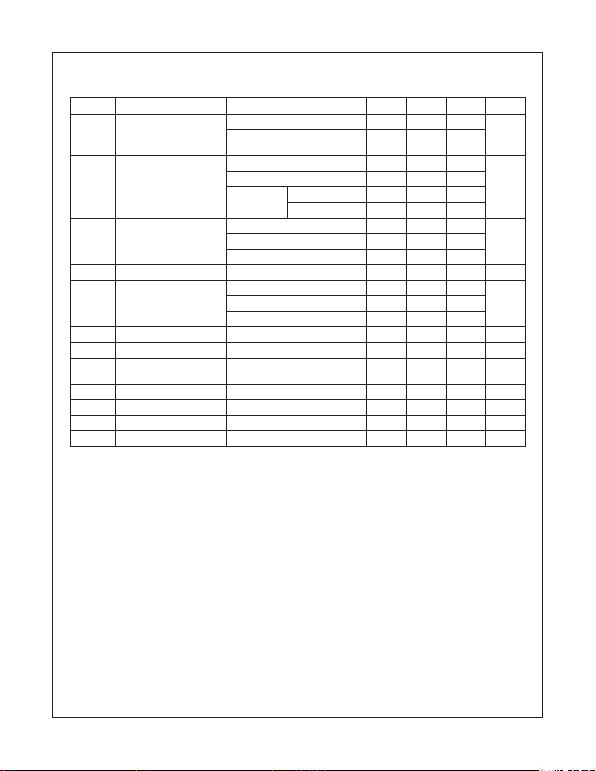14 www.fairchildsemi.com
LM78XX/LM78XXA Rev. 1.0.1
LM78XX/LM78XXA 3-Terminal 1A Positive Voltage Regulator
Electrical Characteristics (LM7808A) (Continued)
Refer to the test circuits. 0°C < TJ < 125°C, IO = 1A, VI = 14V, CI = 0.33µF, C O = 0.1µF, unless otherwise speciﬁed.
Notes:
23. Load and line regulation are speciﬁed at constant junction temperature. Changes in VO due to heating effects must
be taken into account separately. Pulse testing with low duty is used.
24. These parameters, although guaranteed, are not 100% tested in production.
Symbol Parameter Conditions Min. Typ. Max. Unit
VOOutput Voltage TJ = +25°C 7.84 8.0 8.16 V
IO = 5mA to 1A, PO 15W,
VI = 10.6V to 23V
7.7 8.0 8.3
Regline Line Regulation(23) VI = 10.6V to 25V, IO = 500mA 6.0 80.0 mV
VI = 11V to 17V 3.0 80.0
TJ = +25°C VI = 10.4V to 23V 6.0 80.0
VI = 11V to 17V 2.0 40.0
Regload Load Regulation(23) TJ = +25°C, IO = 5mA to 1.5A 12.0 100 mV
IO = 5mA to 1A 12.0 100
IO = 250mA to 750mA 5.0 50.0
IQQuiescent Current TJ = +25°C 5.0 6.0 mA
IQQuiescent Current Change IO = 5mA to 1A 0.5 mA
VI = 11V to 25V, IO = 500mA 0.8
VI = 10.6V to 23V, TJ = +25°C 0.8
VO/TOutput Voltage Drift(24) IO = 5mA -0.8 mV/°C
VNOutput Noise Voltage f = 10Hz to 100kHz, T
A = +25°C 10.0 µV/VO
RR Ripple Rejection(24) f = 120Hz, IO = 500mA,
VI = 11.5V to 21.5V
62.0 dB
VDROP Dropout Voltage IO = 1A, TJ = +25°C 2.0 V
rOOutput Resistance(24) f = 1kHz 18.0 m
ISC Short Circuit Current VI = 35V, TA = +25°C 250 mA
IPK Peak Current(24) TJ = +25°C 2.2 A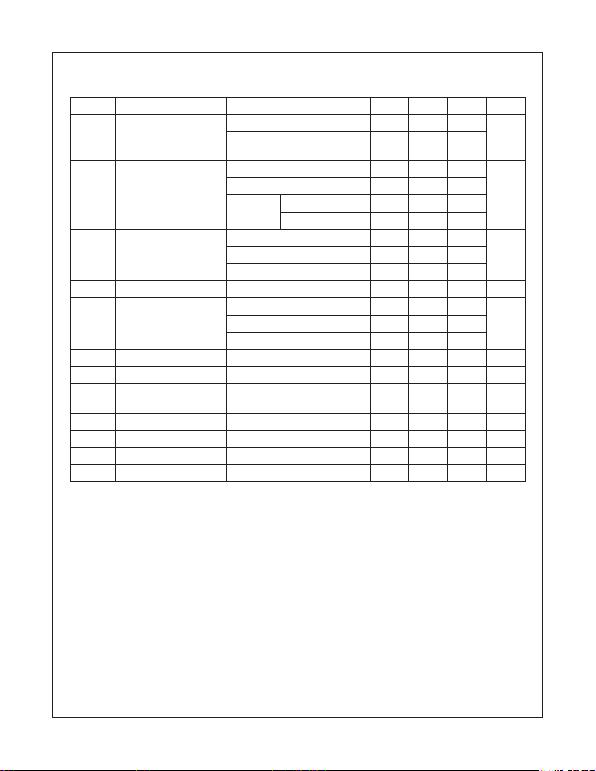15 www.fairchildsemi.com
LM78XX/LM78XXA Rev. 1.0.1
LM78XX/LM78XXA 3-Terminal 1A Positive Voltage Regulator
Electrical Characteristics (LM7809A) (Continued)
Refer to the test circuits. 0°C < TJ < 125°C, IO = 1A, VI = 15V, CI = 0.33µF, C O = 0.1µF, unless otherwise speciﬁed.
Notes:
25. Load and line regulation are speciﬁed at constant junction temperature. Changes in VO due to heating effects must
be taken into account separately. Pulse testing with low duty is used.
26. These parameters, although guaranteed, are not 100% tested in production.
Symbol Parameter Conditions Min. Typ. Max. Units
VOOutput Voltage TJ = +25°C 8.82 9.0 9.16 V
IO = 5mA to 1A, PO 15W,
VI = 11.2V to 24V
8.65 9.0 9.35
Regline Line Regulation(25) VI = 11.7V to 25V, IO = 500mA 6.0 90.0 mV
VI = 12.5V to 19V 4.0 45.0
TJ = +25°C VI = 11.5V to 24V 6.0 90.0
VI = 12.5V to 19V 2.0 45.0
Regload Load Regulation(25) TJ = +25°C, IO = 5mA to 1.5A 12.0 100 mV
IO = 5mA to 1A 12.0 100
IO = 250mA to 750mA 5.0 50.0
IQQuiescent Current TJ = +25°C 5.0 6.0 mA
IQQuiescent Current Change IO = 5mA to 1A 0.5 mA
VI = 12V to 25V, IO = 500mA 0.8
VI = 11.7V to 25V, TJ = +25°C 0.8
VO/TOutput Voltage Drift(26) IO = 5mA -1.0 mV/°C
VNOutput Noise Voltage f = 10Hz to 100kHz, T
A = +25°C 10.0 µV/VO
RR Ripple Rejection(26) f = 120Hz, IO = 500mA,
VI = 12V to 22V
62.0 dB
VDROP Dropout Voltage IO = 1A, TJ = +25°C 2.0 V
rOOutput Resistance(26) f = 1kHz 17.0 m
ISC Short Circuit Current VI = 35V, TA = +25°C 250 mA
IPK Peak Current(26) TJ = +25°C 2.2 A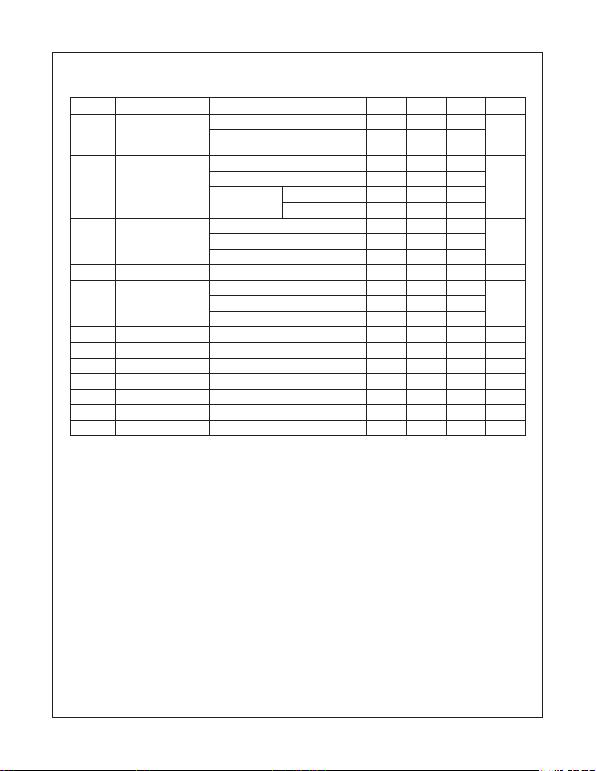16 www.fairchildsemi.com
LM78XX/LM78XXA Rev. 1.0.1
LM78XX/LM78XXA 3-Terminal 1A Positive Voltage Regulator
Electrical Characteristics (LM7810A) (Continued)
Refer to the test circuits. 0°C < TJ < 125°C, IO = 1A, VI = 16V, CI = 0.33µF, C O = 0.1µF, unless otherwise speciﬁed.
Notes:
27. Load and line regulation are speciﬁed at constant junction temperature. Changes in VO due to heating effects must
be taken into account separately. Pulse testing with low duty is used.
28. These parameters, although guaranteed, are not 100% tested in production.
Symbol Parameter Conditions Min. Typ. Max. Units
VOOutput Voltage TJ = +25°C 9.8 10.0 10.2 V
IO = 5mA to 1A, PO 15W,
VI = 12.8V to 25V
9.6 10.0 10.4
Regline Line Regulation(27) VI = 12.8V to 26V, IO = 500mA 8.0 100 mV
VI = 13V to 20V 4.0 50.0
TJ = +25°C VI = 12.5V to 25V 8.0 100
VI = 13V to 20V 3.0 50.0
Regload Load Regulation(27) TJ = +25°C, IO = 5mA to 1.5A 12.0 100 mV
IO = 5mA to 1A 12.0 100
IO = 250mA to 750mA 5.0 50.0
IQQuiescent Current TJ = +25°C 5.0 6.0 mA
IQQuiescent Current
Change
IO = 5mA to 1A 0.5 mA
VI = 12.8V to 25V, IO = 500mA 0.8
VI = 13V to 26V, TJ = +25°C 0.5
VO/TOutput Voltage Drift(28) IO = 5mA -1.0 mV/°C
VNOutput Noise Voltage f = 10Hz to 100kHz, T
A = +25°C 10.0 µV/VO
RR Ripple Rejection(28) f = 120Hz, IO = 500mA, VI = 14V to 24V 62.0 dB
VDROP Dropout Voltage IO = 1A, TJ = +25°C 2.0 V
rOOutput Resistance(28) f = 1kHz 17.0 m
ISC Short Circuit Current VI = 35V, TA = +25°C 250 mA
IPK Peak Current(28) TJ = +25°C 2.2 A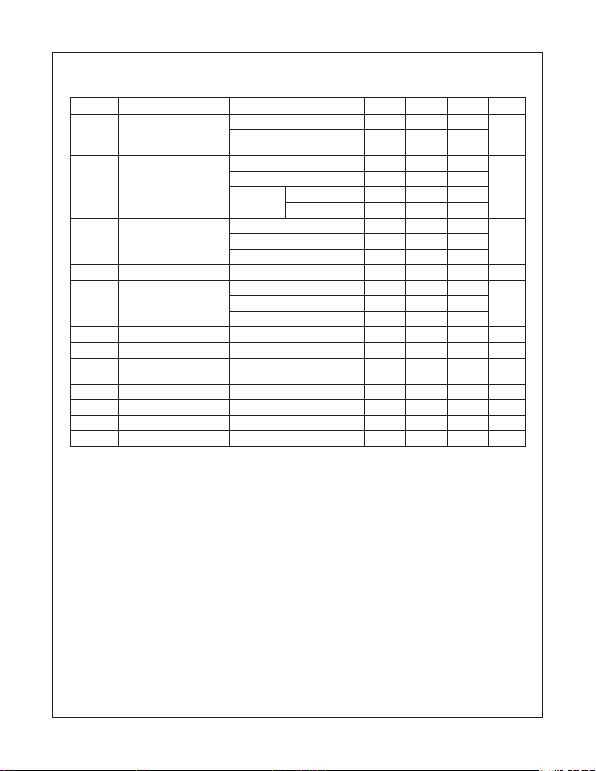17 www.fairchildsemi.com
LM78XX/LM78XXA Rev. 1.0.1
LM78XX/LM78XXA 3-Terminal 1A Positive Voltage Regulator
Electrical Characteristics (LM7812A) (Continued)
Refer to the test circuits. 0°C < TJ < 125°C, IO = 1A, VI = 19V, CI = 0.33µF, C O = 0.1µF, unless otherwise speciﬁed.
Note:
29. Load and line regulation are speciﬁed at constant junction temperature. Changes in VO due to heating effects must
be taken into account separately. Pulse testing with low duty is used.
30. These parameters, although guaranteed, are not 100% tested in production.
Symbol Parameter Conditions Min. Typ. Max. Units
VOOutput Voltage TJ = +25°C 11.75 12.0 12.25 V
IO = 5mA to 1A, PO 15W,
VI = 14.8V to 27V
11.5 12.0 12.5
Regline Line Regulation(29) VI = 14.8V to 30V, IO = 500mA 10.0 120 mV
VI = 16V to 22V 4.0 120
TJ = +25°C VI = 14.5V to 27V 10.0 120
VI = 16V to 22V 3.0 60.0
Regload Load Regulation(29) TJ = +25°C, IO = 5mA to 1.5A 12.0 100 mV
IO = 5mA to 1A 12.0 100
IO = 250mA to 750mA 5.0 50.0
IQQuiescent Current TJ = +25°C 5.1 6.0 mA
IQQuiescent Current Change IO = 5mA to 1A 0.5 mA
VI = 14V to 27V, IO = 500mA 0.8
VI = 15V to 30V, TJ = +25°C 0.8
VO/TOutput Voltage Drift(30) IO = 5mA -1.0 mV/°C
VNOutput Noise Voltage f = 10Hz to 100kHz, T
A = +25°C 10.0 µV/VO
RR Ripple Rejection(30) f = 120Hz, IO = 500mA,
VI = 14V to 24V
60.0 dB
VDROP Dropout Voltage IO = 1A, TJ = +25°C 2.0 V
rOOutput Resistance(30) f = 1kHz 18.0 m
ISC Short Circuit Current VI = 35V, TA = +25°C 250 mA
IPK Peak Current(30) TJ = +25°C 2.2 A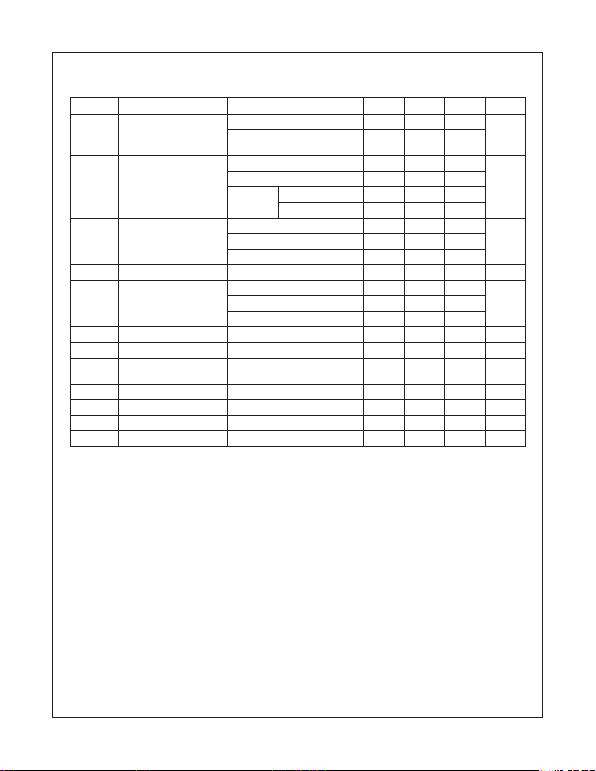18 www.fairchildsemi.com
LM78XX/LM78XXA Rev. 1.0.1
LM78XX/LM78XXA 3-Terminal 1A Positive Voltage Regulator
Electrical Characteristics (LM7815A) (Continued)
Refer to the test circuits. 0°C < TJ < 125°C, IO = 1A, VI = 23V, CI = 0.33µF, C O = 0.1µF, unless otherwise speciﬁed.
Notes:
31. Load and line regulation are speciﬁed at constant junction temperature. Changes in VO due to heating effects must
be taken into account separately. Pulse testing with low duty is used.
32. These parameters, although guaranteed, are not 100% tested in production.
Symbol Parameter Conditions Min. Typ. Max. Units
VOOutput Voltage TJ = +25°C 14.75 15.0 15.3 V
IO = 5mA to 1A, PO 15W,
VI = 17.7V to 30V
14.4 15.0 15.6
Regline Line Regulation(31) VI = 17.4V to 30V, IO = 500mA 10.0 150 mV
VI = 20V to 26V 5.0 150
TJ = +25°C VI = 17.5V to 30V 11.0 150
VI = 20V to 26V 3.0 75.0
Regload Load Regulation(31) TJ = +25°C, IO = 5mA to 1.5A 12.0 100 mV
IO = 5mA to 1A 12.0 100
IO = 250mA to 750mA 5.0 50.0
IQQuiescent Current TJ = +25°C 5.2 6.0 mA
IQQuiescent Current Change IO = 5mA to 1A 0.5 mA
VI = 17.5V to 30V, IO = 500mA 0.8
VI = 17.5V to 30V, TJ = +25°C 0.8
VO/TOutput Voltage Drift(32) IO = 5mA -1.0 mV/°C
VNOutput Noise Voltage f = 10Hz to 100kHz, T
A = +25°C 10.0 µV/VO
RR Ripple Rejection(32) f = 120Hz, IO = 500mA,
VI = 18.5V to 28.5V
58.0 dB
VDROP Dropout Voltage IO = 1A, TJ = +25°C 2.0 V
rOOutput Resistance(32) f = 1kHz 19.0 m
ISC Short Circuit Current VI = 35V, TA = +25°C 250 mA
IPK Peak Current(32) TJ = +25°C 2.2 A